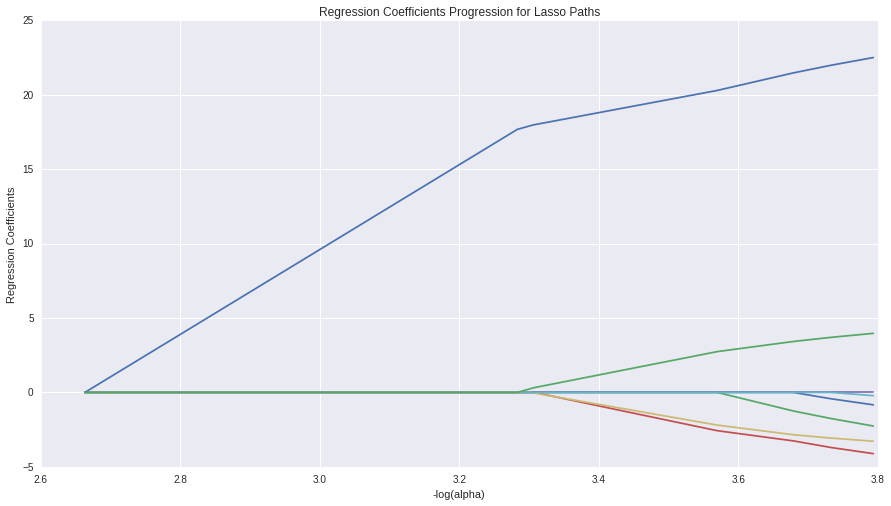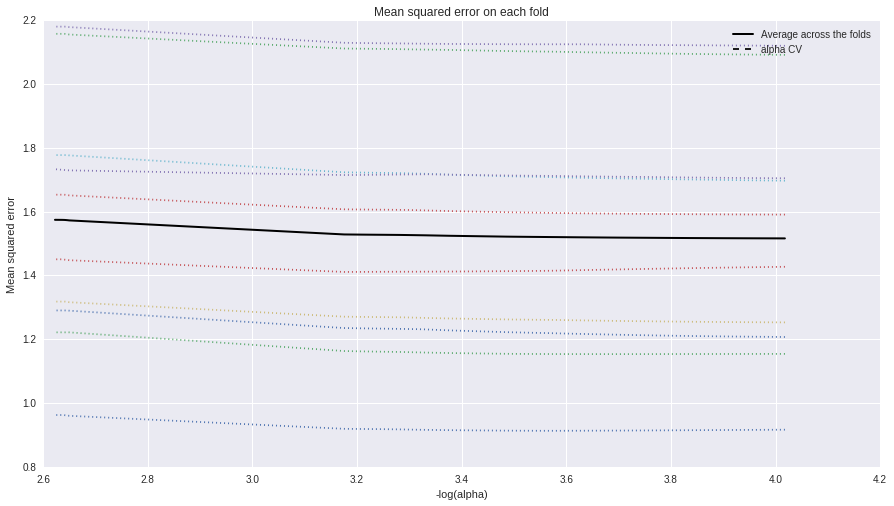# samucoder

Samuel M.H. 's technological blog

Notebook

# Running a Lasso Regression Analysis

Author: Samuel M.H. <samuel.mh@gmail.com> Date: 04-03-2016

# Instructions

This week’s assignment involves running a lasso regression analysis. Lasso regression analysis is a shrinkage and variable selection method for linear regression models. The goal of lasso regression is to obtain the subset of predictors that minimizes prediction error for a quantitative response variable. The lasso does this by imposing a constraint on the model parameters that causes regression coefficients for some variables to shrink toward zero. Variables with a regression coefficient equal to zero after the shrinkage process are excluded from the model. Variables with non-zero regression coefficients variables are most strongly associated with the response variable. Explanatory variables can be either quantitative, categorical or both.

Your assignment is to run a lasso regression analysis using k-fold cross validation to identify a subset of predictors from a larger pool of predictor variables that best predicts a quantitative response variable.

## What to Submit

Following completion of the steps described above, create a blog entry where you submit syntax used to run a lasso regression (copied and pasted from your program) along with corresponding output and a brief written summary. Please note that your reviewers should NOT be required to download any files in order to complete the review.

If your data set has a relatively small number of observations, you do not need to split into training and test data sets. You can provide your rationale for not splitting your data set in your written summary.

## Intro

This week I will try to explain the average daily volume of ethanol consumed per person in past year with a LASSO regression model.

## Dataset

### Variables

• Response:

• ETOTLCA2 -> ETHANOL: average daily volume of ethanol consumed in past year (ounces).
• Explanatory:

• AGE -> AGE: age (years).
• S1Q24LB -> WEIGHT: weight (pounds).
• NUMPERS -> HOUSE_PEOPLE: number of persons in household.
• S1Q4A -> MARRAIGE: age at first marriage (years).
• S1Q8D -> WORK: age when first worked full time, 30+ hours a week (years).
• S1Q12A -> INCOME: total household income in last 12 months (dolars).
• SEX -> MALE: gender (2 groups).
• S10Q1A63 -> CHANGE_MIND: change mind about things depending on people you're with or what read or saw on tv (2 groups).

All used variables are quantitative.

In :
%pylab inline

import numpy as np
import pandas as pd

from sklearn.cross_validation import train_test_split
import sklearn.metrics
from sklearn.linear_model import LassoLarsCV

#Visualization
import matplotlib.pylab as plt
import seaborn as sns

pylab.rcParams['figure.figsize'] = (15, 8)

Populating the interactive namespace from numpy and matplotlib


WARNING: pylab import has clobbered these variables: ['plt']
%matplotlib prevents importing * from pylab and numpy



## Data

In :
# Load data

In :
# Custom dataframe
df = pd.DataFrame()

# Response variable
df['ETHANOL'] = data['ETOTLCA2'].replace(' ',np.NaN).astype(float)

# Explanatory variables
df['AGE'] = data['AGE'].replace(' ',np.NaN).replace('98',np.NaN).astype(float)
df['WEIGHT'] = data['S1Q24LB'].replace(' ',np.NaN).replace('999',np.NaN).astype(float)
df['HOUSE_PEOPLE'] = data['NUMPERS'].replace(' ',np.NaN).astype(float)
df['MARRIAGE'] = data['S1Q4A'].replace(' ',np.NaN).replace('99',np.NaN).astype(float)
df['WORK'] = data['S1Q8D'].replace(' ',np.NaN).replace('99',np.NaN).replace('0',np.NaN).astype(float)
df['INCOME'] = data['S1Q12A'].replace(' ',np.NaN).astype(float)
df['MALE'] = data['SEX'].replace(' ',np.NaN).replace('2','0').astype(float)
df['CHANGE_MIND'] = data['S10Q1A63'].replace(' ',np.NaN).replace('9',np.NaN).replace('2','0').astype(float)

df = df.dropna()
df.describe()

Out:
ETHANOL AGE WEIGHT HOUSE_PEOPLE MARRIAGE WORK INCOME MALE CHANGE_MIND
count 15307.000000 15307.000000 15307.000000 15307.000000 15307.000000 15307.000000 15307.000000 15307.000000 15307.000000
mean 0.490314 45.146338 174.669628 2.736199 23.537532 19.021297 62375.538642 0.503234 0.148559
std 1.211545 13.486408 40.473162 1.422247 5.078884 4.496707 70392.218489 0.500006 0.355665
min 0.000300 18.000000 78.000000 1.000000 14.000000 5.000000 24.000000 0.000000 0.000000
25% 0.016800 35.000000 145.000000 2.000000 20.000000 17.000000 29999.000000 0.000000 0.000000
50% 0.103700 43.000000 170.000000 2.000000 23.000000 18.000000 50000.000000 1.000000 0.000000
75% 0.475450 54.000000 198.500000 4.000000 26.000000 21.000000 76000.000000 1.000000 0.000000
max 29.676500 94.000000 450.000000 13.000000 63.000000 71.000000 3000000.000000 1.000000 1.000000
In :
TARGET = 'ETHANOL'
PREDICTORS = list(df.columns)
PREDICTORS.remove(TARGET)

df_target = df[TARGET]
df_predictors = pd.DataFrame()


### Standardize predictors

• 0 Mean
In :
for predictor in PREDICTORS:
pred_data = df[predictor]
df_predictors[predictor] = (df[predictor] - df[predictor].mean()) / df[predictor].std()

In :
df_predictors.describe()

Out:
AGE WEIGHT HOUSE_PEOPLE MARRIAGE WORK INCOME MALE CHANGE_MIND
count 1.530700e+04 1.530700e+04 1.530700e+04 1.530700e+04 1.530700e+04 1.530700e+04 1.530700e+04 1.530700e+04
mean -1.290461e-16 -5.756014e-17 -6.127369e-17 1.559694e-16 -8.541181e-17 -1.114067e-17 7.519953e-17 4.270591e-17
std 1.000000e+00 1.000000e+00 1.000000e+00 1.000000e+00 1.000000e+00 1.000000e+00 1.000000e+00 1.000000e+00
min -2.012866e+00 -2.388487e+00 -1.220744e+00 -1.877880e+00 -3.118126e+00 -8.857732e-01 -1.006456e+00 -4.176946e-01
25% -7.523381e-01 -7.330692e-01 -5.176310e-01 -6.965176e-01 -4.495061e-01 -4.599449e-01 -1.006456e+00 -4.176946e-01
50% -1.591483e-01 -1.153759e-01 -5.176310e-01 -1.058366e-01 -2.271212e-01 -1.758083e-01 9.935207e-01 -4.176946e-01
75% 6.564878e-01 5.887944e-01 8.885945e-01 4.848444e-01 4.400337e-01 1.935507e-01 9.935207e-01 -4.176946e-01
max 3.622437e+00 6.802789e+00 7.216609e+00 7.769910e+00 1.155928e+01 4.173223e+01 9.935207e-01 2.393937e+00

### Split: train, test

In :
train_target, test_target, train_predictors, test_predictors = train_test_split(df_target, df_predictors, test_size=0.3, random_state=42)

print('Samples train: {0}'.format(len(train_target)))
print('Samples test:  {0}'.format(len(test_target)))

Samples train: 10714
Samples test:  4593



## Model

In :
model1 = LassoLarsCV(cv=10,precompute=False)
model1.fit(train_predictors, train_target)

Out:
LassoLarsCV(copy_X=True, cv=10, eps=2.2204460492503131e-16,
fit_intercept=True, max_iter=500, max_n_alphas=1000, n_jobs=1,
normalize=True, positive=False, precompute=False, verbose=False)

In :
print('Alpha parameter: {0}'.format(model1.alpha))

Alpha parameter: 0.0



### Regression coeficients

In :
coefs = zip(df_predictors.columns,model1.coef_)
coefs.sort(key=lambda x: abs(x), reverse=True)
print '\n'.join( '{0}: {1}'.format(var,coef) for var,coef in coefs)

MALE: 0.25510029979
HOUSE_PEOPLE: -0.0664057742071
CHANGE_MIND: 0.0554624412918
WEIGHT: -0.0550868686148
WORK: -0.0437114356411
AGE: -0.0349338129742
MARRIAGE: -0.0195949134257
INCOME: -0.0138293582608



### Plots

In :
# plot coefficient progression
m_log_alphas = -np.log10(model1.alphas_)
ax = plt.gca()
plt.plot(m_log_alphas, model1.coef_path_.T)
plt.axvline(-np.log10(model1.alpha_), linestyle='--', color='k',label='alpha CV')
plt.ylabel('Regression Coefficients')
plt.xlabel('-log(alpha)')
plt.title('Regression Coefficients Progression for Lasso Paths')

Out:
<matplotlib.text.Text at 0x7fc86aa34550>In :
# plot mean square error for each fold
m_log_alphascv = -np.log10(model1.cv_alphas_)
plt.figure()
plt.plot(m_log_alphascv, model1.cv_mse_path_, ':')
plt.plot(m_log_alphascv, model1.cv_mse_path_.mean(axis=-1), 'k', label='Average across the folds', linewidth=2)
plt.axvline(-np.log10(model1.alpha_), linestyle='--', color='k', label='alpha CV')
plt.legend()
plt.xlabel('-log(alpha)')
plt.ylabel('Mean squared error')
plt.title('Mean squared error on each fold')

Out:
<matplotlib.text.Text at 0x7fc86a8669d0>### Metrics

In :
# MSE from training and test data
print ('MSE training: {0}'.format(sklearn.metrics.mean_squared_error(train_target, model1.predict(train_predictors))))
print ('MSE testing: {0}'.format(sklearn.metrics.mean_squared_error(test_target, model1.predict(test_predictors))))

MSE training: 1.51194970787
MSE testing: 1.15947090575


In :
# R-square from training and test data
print ('R-square training: {0}'.format(model1.score(train_predictors,train_target)))
print ('R-square testing: {0}'.format(model1.score(test_predictors,test_target)))

R-square training: 0.0396962849816
R-square testing: 0.048380464543



## Summary

In this assignment, the LASSO regression hasn't proved to be very valuable as the model can only explain a 4,8% of the variance (R-Squared value). It is surprising this value is higher in the test dataset than in the training one. It happens the same with the Mean Squared Error, it is lower in the testing dataset.

The prediction accuracy is pretty stable as the metric values are similar in training and testing datasets.

The most important variable to predict the alcohol ingest is MALE: 0.25510029979, followed by HOUSE_PEOPLE: -0.0664057742071 CHANGE_MIND: 0.0554624412918, WEIGHT: -0.0550868686148 and WORK: -0.0437114356411. Surprisingly, the income is not as relevant as the others but it is not discarded.

One bad thing about the results is getting an Alpha value of 0, this means no regularization has been done and an Ordinary Least Squares regression has been performed. This can be verified as no predictor has been discarded, every one has a coefficient bigger than one.

The LASSO regression is useful when there are few observations and a large number of predictors so it can be used for dimensionality reduction.